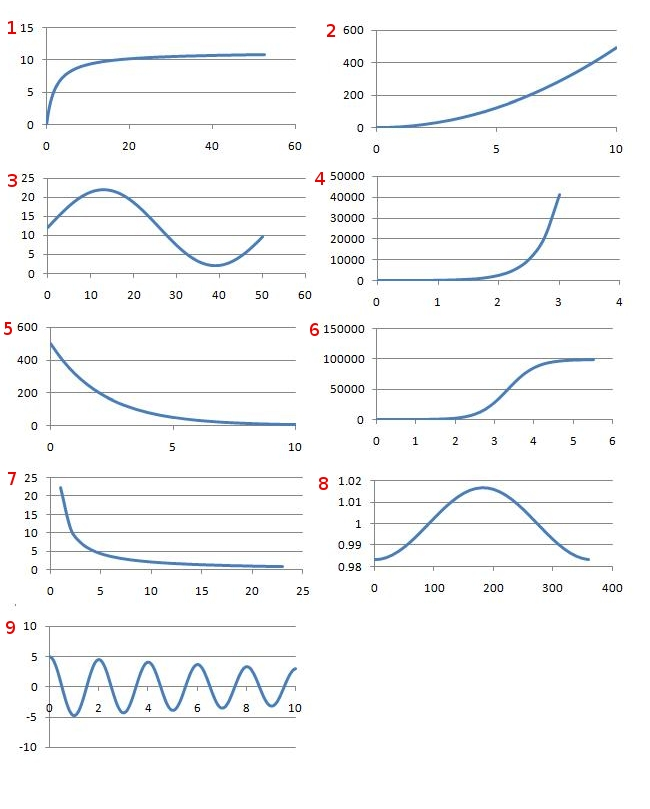#### You may also like### How Many Solutions?

Find all the solutions to the this equation.### Quartics

Investigate the graphs of y = [1 + (x - t)^2][1 + (x + t^)2] as the parameter t varies.### Power Up

Show without recourse to any calculating aid that 7^{1/2} + 7^{1/3} + 7^{1/4} < 7 and 4^{1/2} + 4^{1/3} + 4^{1/4} > 4 . Sketch the graph of f(x) = x^{1/2} + x^{1/3} + x^{1/4} -x

# Whose Line Graph Is it Anyway?

##### Age 16 to 18Challenge LevelProcess Graph Equation Explanation 1 6 H In a food-limited environment bacteria tend to maximum number after initial exponential growth (following lag phase) 2 5 B Concentration will exponentially decay as drug is metabolised 3 9 E Pendulum will have an sinusodial motion with a decaying amplitude due to damping from air resistance 4 7 I Ideal gas equation $pV = nRT$ therefore pressure and volume are in a reciprocal relationship 5 2 A Ball is small and heavy, therefore assume air resistance is negligible at first and so acceleration is constant. $s = ut + \frac{1}{2}at^2$ therefore for $u=0;\ a=g \Rightarrow s=\frac{1}{2}gt^2$ (qualitatively vertical speed represented by gradient of graph is increasing) 6 1 F As concentration of reagent increases so does reaction rate, however as increase continues concentration of the catalyst becomes the limiting factor (saturation) 7 4 G When not food limited, bacteria follow exponential growth after initial lag phase 8 3 D Hours of daylight varies from a maximum at mid-summer to minimum at mid-winter, with a mean value of 12 9 8 C Earth's orbit is not perfectly circular therefore small oscillations about mean distance (1 AU) with period of one year (note non-zero origin on vertical axis)